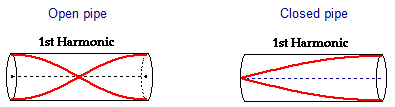# Waves in a closed organ pipe (homework check)

Sullo

## Homework Statement

A closed organ pipe of length 1.00m is filled with a gas and is found to give the same note as an open pipe of length 1.30m filled with air, when both are resonating at their fundamental mode of vibration

a) draw diagrams to show the nature of the waves in each pipe and use them to assist in your working
b) find the ratio of the velocity of sound in the gas compared to the velocity of sound in air.

b)
f(open) = v/2L
f(closed) = v/4L

## The Attempt at a Solution

a) The diagrams i've drawn look like this:

open:https://imgur.com/a/MXcmJ5h closed: https://imgur.com/a/cK79JjLb)
f(open) = v/2L
v = 1/2Lf
-> 1/2(1.30)f
=1/2.60f

f(closed) = v/4L
v = 1/4Lf
v = 1/4(1.00)f
v = 1/4.00f

ratio = vclosed/vopen

= (1/4.00f) / (1/2.60f)
= 2.60f/4.00f
= 2.60/4.00 (f cancels, same pitch)
= 0.650

#### Attachments

Last edited by a moderator:

Mentor
b)
f(open) = v/2L
v = 1/2Lf
-> 1/2(1.30)f
=1/2.60f

f(closed) = v/4L
v = 1/4Lf
v = 1/4(1.00)f
v = 1/4.00f
Check your algebra for both: you've made the same mistake rearranging the expressions to solve for v in each case.

Sullo
Check your algebra for both: you've made the same mistake rearranging the expressions to solve for v in each case.
Thank you for pointing this out.

So it should be v = 2lf v = 4lf ?

Mentor
Thank you for pointing this out.

So it should be v = 2lf v = 4lf ?
Yes.

I might also suggest that you alter the variable names to distinguish the two cases right from the start. This can help prevent mistakenly combining or cancelling variables that really are different when you work the algebra (Not that you've done this here, I'm just saying for future work where the equations might be more complicated). It will also make your work more clear and easy to follow for others.

It's clear that there are two different lengths and velocities involved in the problem, one for the open pipe and one for the closed pipe. Why not write them as ##L_o## and ##v_o##, and ##L_c## and ##v_c## for example?